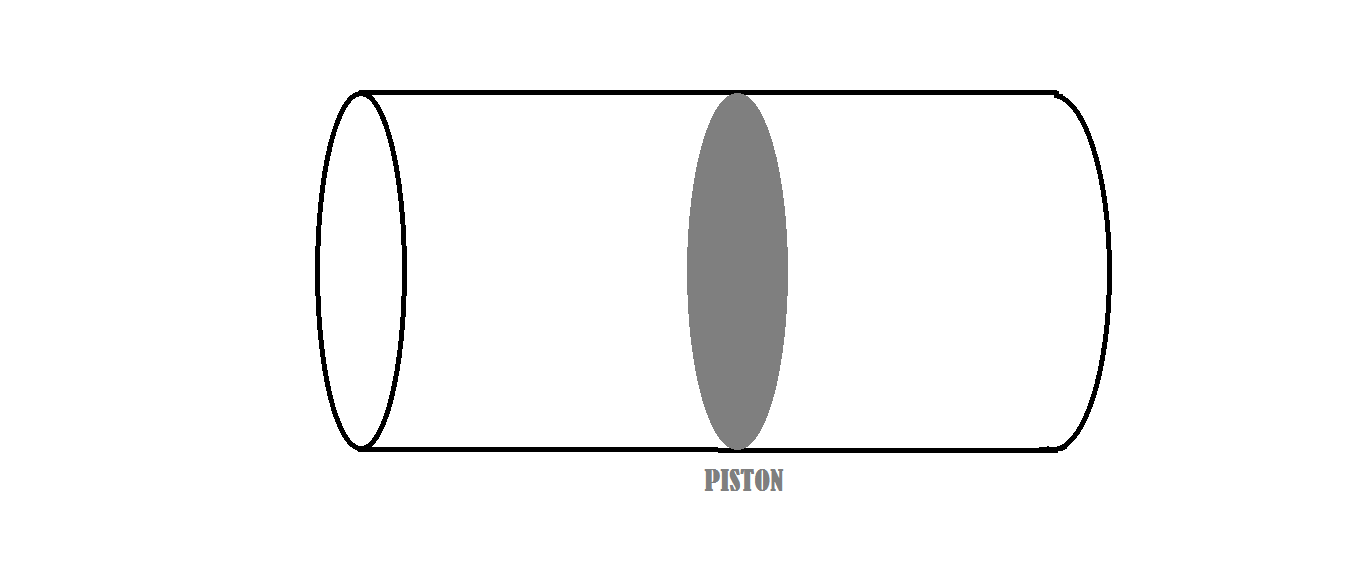# Work In Thermodynamic ProcessA piston is placed inside a cylindrical container, which has adiabatic walls. Initially, the piston divides the container into two equal parts, with volumes $V$ each, both containing an ideal gas $G$ at a pressure $P$. Then the piston is moved very slowly by an external force $F_{ext}$ till the volume on one side of the piston becomes thrice that on the other side.

Let the work done by $F_{ext}$ in doing so be $W$. $W=kPV$

If the heat capacity ratio of $G$ is $\dfrac{5}{3}$, then find $k$.

Note : Take the piston to be insulating.

×

Problem Loading...

Note Loading...

Set Loading...